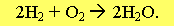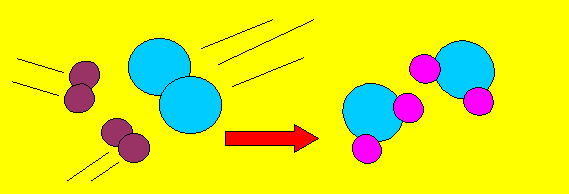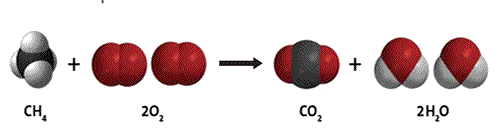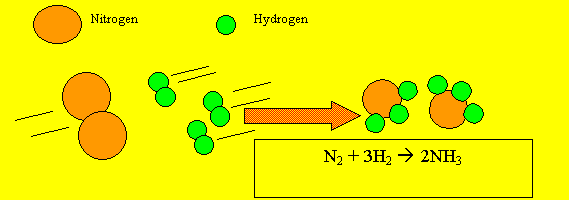Chemical Equations Scientists use a special way of writing to describe what happens in a chemical reaction. They describe a chemical reaction by writing a chemical equation using chemical symbols to represent the atoms taking part. Below is an example of a chemical equation using chemical formulae instead of words..This equation tells us that two molecules of hydrogen gas react with one molecule of oxygen gas to produce two molecules of water. The equation has two sides. On the left of the arrow we put the reactants(the chemicals that react together). On the right side of the arrow we put the products (the chemicals) that are formed or produced. If we were to draw this reaction in pictures it would look like this.We can see that two molecules of hydrogen react with one molecule of oxygen to produce two molecules of water Why do we have a 2 in front of the water and the hydrogen molecule in the equation? This is called "BALANCING the equation". There is a special Law in Nature that states that "Matter can not be created or destroyed". It is called "The Law Of Conservation Of Mass". Click to see a demonstration of this law. What this means is that we have to have the same number of each atom on each side of the arrow in the equation. Methane and oxygen react to form carbon dioxide and water CH4 + 2O2 => CO2 + 2H2O The equation reveals that 1 2 3 4 5 molecule/s of methane react/s with 1 2 3 4 5 molecule/s of oxygen to form 1 2 3 4 5 molecule/s of carbon dioxide and 1 2 3 4 5 molecule/s of water. How many oxygen atoms on the right of the equation 1 2 3 4 5 How many oxygen atoms on the left of the equation 1 2 3 4 5 How many hydrogen atoms on the right of the equation 1 2 3 4 5 How many hydrogen atoms on the left of the equation 1 2 3 4 5 How many carbon atoms on the right of the equation 1 2 3 4 5 How many carbon atoms on the leftt of the equation 1 2 3 4 5Write an equation for each of the reactions on the next page. Here is an exampleGo to the reactions